# CBSE Important Questions

## Mathmatics Class IX

Q. 1. Factorize the following :

1. 49a2 + 70ab + 25b2
2.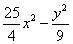Q. 2. (a) Find the zeroes of the polynomial p(x) = 2x + 1

(b) Check whether -2 & 2 are the zeroes of polynomial x + 2 or not .

Q. 3. Expand the following :-

1. (-2x + 5y – 32)2
2.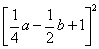Q. 4. Verify that x3 + y3 + z3 – 3xyz =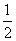(x+y+z)[(x-y)2 + (y-z)2 + (z-x)2]

Q. 5. Factorize :

(i) x3 + 13x2 + 32x + 20 (ii) 2y3 + y2 – 2y – 1
(iii)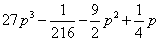Q. 6. (a) Evaluate : (998)3

1. Without calculating the cube, find the value of (28)3 + (-15)3 + (-13)3

Q. 7. If x+ y + z = 0, then show that x3 + y3 + z3 = 3xyz.

Q. 8. Factorize the following using factor theorem :

1. y2 – 5y + 6
2. x3 – 23x2 + 142 x – 120

Q. 9. (a) Find the value of k, if x -1 is a factor of p(x) where p(x) = 2x2 + kx +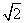1. Find the remainder when x3 – 9x2 + 6x –a is divided by x – a .

Q. 10. (a). Find p(0), p(1) & p(2) for the following polynomial :

1. p(t) = 2 + t + 2t2 – t3

(b). Find the coefficient of x2 in the following polynomial as well as its degree

1. 6x – 7x3 + 8x4 + 3x2
2. 2 – x2 – y3 + 2y8